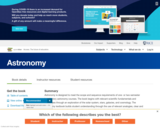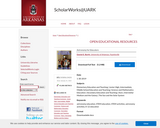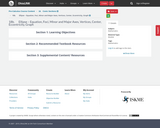Updating search results...

# 4 Results

View
Selected filters:
• AstronomyUnrestricted Use
CC BY
Rating
0.0 stars

Astronomy is designed to meet the scope and sequence requirements of one- or two-semester introductory astronomy courses. The book begins with relevant scientific fundamentals and progresses through an exploration of the solar system, stars, galaxies, and cosmology. The Astronomy textbook builds student understanding through the use of relevant analogies, clear and non-technical explanations, and rich illustrations. Mathematics is included in a flexible manner to meet the needs of individual instructors.

Subject:
Astronomy
Physical Science
Material Type:
Textbook
Provider:
Rice University
Provider Set:
Openstax College
Author:
Andrew Fraknoi
David Morrison
Sidney C. Wolff
04/27/2020Conditional Remix & Share Permitted
CC BY-SA
Rating
0.0 stars

Astronomy for Educators provides new and accomplished K-12 instructors with concepts and projects for low-cost, high-impact STEM classroom instruction that is built around the National Academies National Research Council's K-12 Framework for Science Education.

Subject:
Astronomy
Physical Science
Material Type:
Textbook
Author:
Daniel E. Barth
01/30/2019Conditional Remix & Share Permitted
CC BY-NC
Rating
0.0 stars

Ellipse - conic section, foci, major and minor axes, vertices, standard form, eccentricityTMM 002 PRECALCULUS (Revised March 21, 2017)AdditionalOptional Learning Outcomes:2. Geometry: The successful Precalculus student can:2f. Represent conic sections algebraically via equations of two variables and graphically by drawing curves.Sample Tasks:The student can perform the process &ldquo;completing the square&rdquo; transforming the equation into a standard form.The student can draw curves representing conic sections.The student can solve systems of equations involving linear and quadratic functions.The student can parametrize conic curves.

Subject:
Algebra
Astronomy
Geometry
Higher Education
Mathematics
Material Type:
Module
05/28/2019Conditional Remix & Share Permitted
CC BY-NC
Rating
0.0 stars

Hyperbola - conic section, foci, transverse and conjugate axes, vertices, asymptotes, standard formTMM 002 PRECALCULUS (Revised March 21, 2017)AdditionalOptional Learning Outcomes:2. Geometry: The successful Precalculus student can:2f. Represent conic sections algebraically via equations of two variables and graphically by drawing curves.Sample Tasks:The student can perform the process &ldquo;completing the square&rdquo; transforming the equation into a standard form.The student can draw curves representing conic sections.The student can solve systems of equations involving linear and quadratic functions.The student can parametrize conic curves.

Subject:
Astronomy
Geometry
Higher Education
Mathematics
Material Type:
Module